Jekyll2023-01-24T08:40:10+00:00https://sdaza.github.io/feed.xmlblankDemography, Sociology, Simulation, Data ScienceExtracting Zotero’s notes2021-07-06T00:00:00+00:002021-07-06T00:00:00+00:00https://sdaza.github.io/blog/2021/zotero-notesWhen reviewing the literature, it’s nice to have the notes linked to Zotero references. It would also be great to compare them systematically. The idea would be to have your notes in Zotero so that everyone in the group can access and edit them and create a data file to explore your notes using, for instance, text analysis techniques.

This small package extracts notes from a collection and creates a CSV file that can be easily read using Excel. You only need to specify the collection ID. For instance, if the location of my collection is https://www.zotero.org/groups/2406179/csic-echo/collections/M8N2VMAP, the collection ID would be M8N2VMAP. We also need the Zotero API’s credentials.

To create a clean CSV, notes’ headers would need a suitable separator. The default is #. In this case, the text between headings mustn’t include #. Below an example:

# Research question

Estimates interaction effects between PGS of obesity and cohorts using HRS.

# Data

HRS

# Methods

Uses a HLM whereby they estimate effects of age and cohorts while making the
intercepts and slopes a function of individual factors.


## Installation

pip install git+https://github.com/sdaza/zotnote.git


## Credentials

You can save your Zotero API credentials in a config.py and load them using import config:

library_id = "0000000"
api_key = "key"
library_type = "group"


## Example

Let’s try to extract some notes and read them using Pandas:

Notes saved in zotero-notes.csv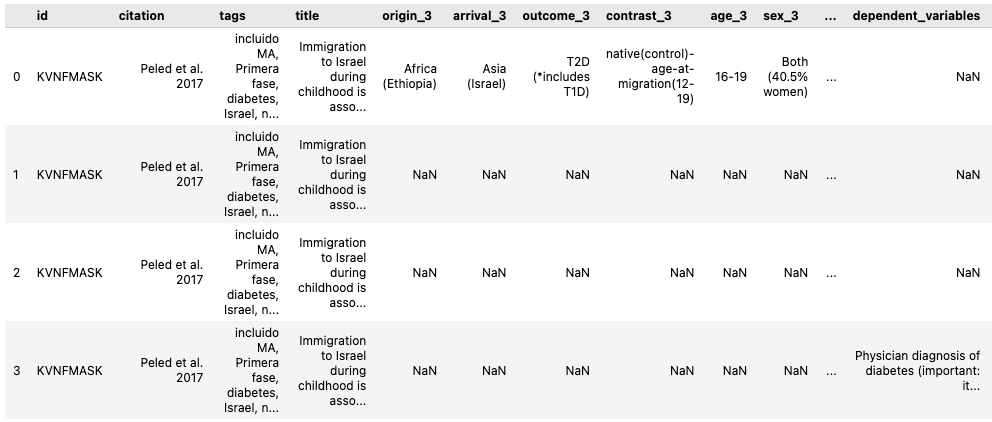]]>
Sebastian Daza
From database to CSV using Anylogic2020-11-03T00:00:00+00:002020-11-03T00:00:00+00:00https://sdaza.github.io/blog/2020/anylogic-databaseWhen running a parameter variation experiment, that is, simulating over several iterations and replicates using parallelization, we usually need to collect a huge amount of data and have them in a format that then we can process using Python or R.

The best way to do this in Anylogic would be using a database and then export, read, or connect to the database to process simulation results (although, see the section update below). We can do that easily in Anylogic. Every time an experiment finishes, we can export the data (from a database) to an Excel file manually.

The issue with Excel files is, on the one hand, they are Excel files, and on the other, they are not suitable for big data (more than 1 million rows). We can create a function to save all the simulation tables into an Excel file as our experiment finishes. However, we will still have the limit-of-rows limitation (check the Anylogic file linked below for a function to create Excel files from a database).

Here I follow a different approach by exporting an Anylogic database table to a CSV file within Java. The general setup using Anylogic PLE 8.6 would be:

• Create the databases you need for your experiment, and be sure you add the columns iteration and replicate.
• Create a function to save the data of your simulation runs (e.g., agent’s status, age, etc.)
• Define a parameter variation experiment.
• Define a variable to specify where to save the data (i.e., path).
• Write code in the experiment Java Actions section so that to save data every time you run an experiment.
• Import functions in the advanced Java section of the experiment.

## Databases

I define two tables (data1 and data2). Each agent saves its data at a given rate. After 5 years, the simulation will finish.

The tables include a column with the experiment iteration and replicate, in addition to the agent’s index, time, and a random value from a normal distribution.

## From DB to CSV

The key function to export the data to a CSV file is f_SQLToCSV. It uses two arguments, a SQL query (query) and the path to an output file (filename). For instance, we can write:

You can use any query for your data, giving you a lot of flexibility on what to export to a CSV file. The f_SQLToCSV method is:

The next step would be to create an experiment and complete the Java actions accordingly. First, we clear our tables.

Then, we collect information on the iteration and replicate of the simulation run:

Finally, at the end of the experiment, we save the data and clear the tables again:

The method f_exportTables is just a function that goes through each table and export them to a CSV file. v_tables is string array with the name of the tables I want to export {"data1", "data2"}:

Remember to import some functions in the imports section:

From there, we can create additional functions to select the tables to be exported. For more details, download the Anylogic File here.

## Update

When running several replicates of my simulation, saving the information in a database didn’t work as expected. My simulation just crashed, and I was not able to keep the data. I finally decided to follow my previous approach: create many CSV files – one per iteration and replicate – and read them using an R or Python function. I know you end up with a lot of CSV files, but at least the simulation doesn’t crash, and you can recover the output of your simulation as it goes.

]]>
Sebastian Daza
Oh, descriptive tables (R + Latex)!2020-05-12T00:00:00+00:002020-05-12T00:00:00+00:00https://sdaza.github.io/blog/2020/descriptive-tablesIt’s been a while since my last post. It’s time to catch up!

Every time I write a paper or report, I need to create descriptive tables using Latex. Over and over I create Adhoc tables, and I say to myself: Write a general function so you can save time in the next paper! I know there are some solutions out there, but in general, I feel they are not flexible enough.

I introduce a far from perfect function to create descriptive tables in Latex. The steps and structure are quite simple:

1. Write a function to summarize your data with any stats you want
2. Define a list with the data plus column names (labels)

That’s it. You can see the function here. It has some features might be useful:

• It deals automatically with factors (categorical variables)
• You can use different datasets at the same time
• You can group columns using a variable (e.g., year)
• You can add long notes at the bottom of the table
• You can specify your own descriptive function

Let’s start creating some fake data:

• 5 variables
• Variable 3 is a factor (i.e., categorical)
• Variable 5 is a grouping column

We can define a descriptive function: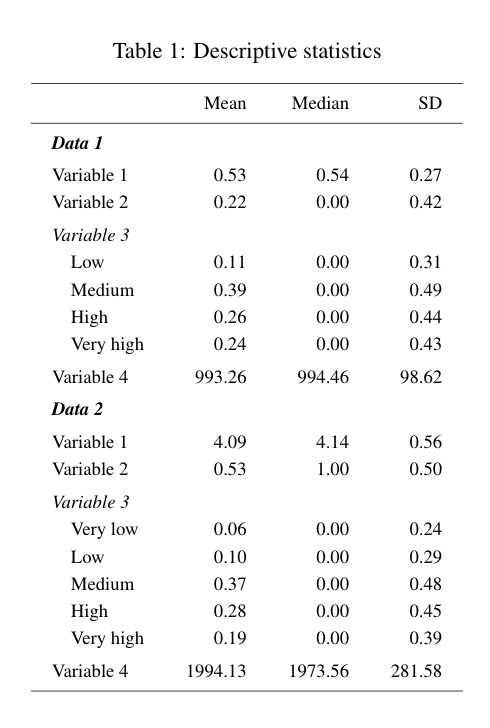Thus, the grouping of rows is defined by the name of each dataset in the list. We can add a note, just remember to add \usepackage[flushleft]{threeparttable} to your Latex document: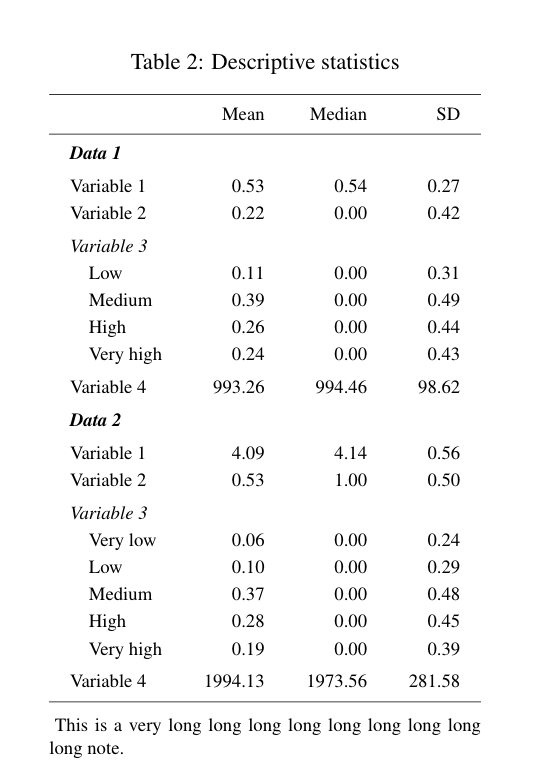We can also slice the descriptives by group: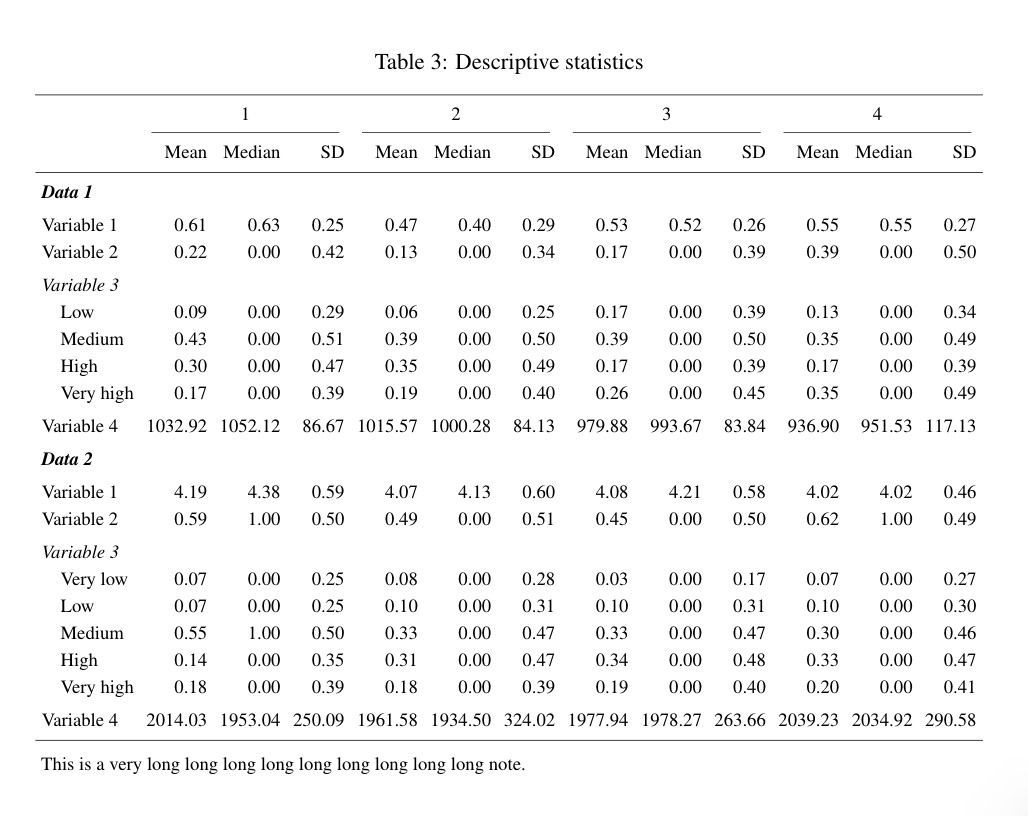It’s just a first version. I will add more features soon.

]]>
Sebastian Daza
Reading CDC mortality files using R2016-10-05T00:00:00+00:002016-10-05T00:00:00+00:00https://sdaza.github.io/blog/2016/read-mortality-dataReading fixed-width text files might be challenging, specially when we don’t have a dictionary file. In this post, I show steps to read CDC files in a more systematic way. In this example, I import a compress mortality file (CMF 1979-1988) available here and whose codebook (or layout) is here.

To read this file, usually with extension .txt or .dat, I first need to know where each column starts and finishes. What I get from the pdf file is something like this: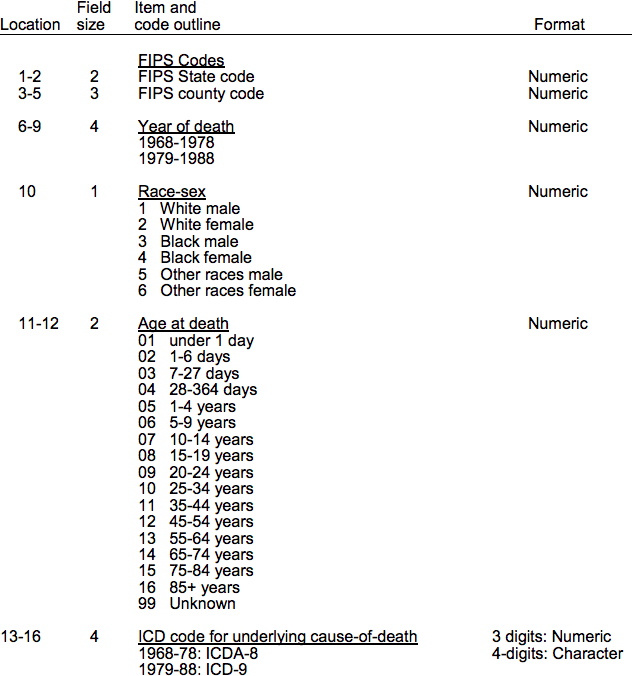The layout is usually a codebook in Word/PDF or just plain text file. Here, I copy the PDF text and put it in a plain text file. I use a text editor (e.g., Sublime Text) and regular expressions to extract the information I need.

I have to select every row with this pattern: 1-2 2 FIPS State code Numeric. That is, a number followed by a hyphen (although not always, particularly when the width of the column is one), spaces, another number, spaces, and then any text. I use the following regular expression to get that pattern: (^[0-9]+).([0-9]+)\s+([0-9])\s+(.+). Using the Sublime package Filter Lines I get something like this (you can also just copy the selected lines):

1-2 2 FIPS State code Numeric
3-5 FIPS county code Numeric
6-9 4 Year of death Numeric
11-12 2 Age at death Numeric
13-16 4 ICD code for underlying cause-of-death 3 digits: Numeric
17-19 3 Cause-of-Death Recode Numeric
20-23 4 Number of deaths Numeric


This approach might be particularly useful when you have a long PDF/Word file and you want to extract most of the variables. You would need to adapt the regular expressions I’m using to the particular patterns of your codebook.

To simplify, I format this text as a comma-separated values file (csv). Replacing this regular expression ([0-9]+)(-)([0-9]+)(\s)([0-9]+)(\s)(.+)(\s)(Numeric) by \1,\3,\5,\7,\9 I get:

1,2,2,FIPS State code,Numeric
3,5,3,FIPS county code,Numeric
6,9,4,Year of death,Numeric
11,12,2,Age at death,Numeric
13,16,4,ICD code for underlying cause-of-death 3 digits:,Numeric
17,19,3,Cause-of-Death Recode,Numeric
20,23,4,Number of deaths,Numeric


Then, I read the layout file:

Now, I can read the fixed-width data file. I use the readr package (in my experience relatively fast for big datasets ~ 1 GB).

Hopefully, this might save you some time!

Last Update: 06/29/2017

]]>
Sebastian Daza
R package to compute statistics from the American Community Survey (ACS) and Decennial US Census2016-07-06T00:00:00+00:002016-07-06T00:00:00+00:00https://sdaza.github.io/blog/2016/acsrThe acsr package helps extracting variables and computing statistics using the America Community Survey and Decennial US Census. It was created for the Applied Population Laboratory (APL) at the University of Wisconsin-Madison.

## Installation

The functions depend on the acs and data.table packages, so it is necessary to install then before using acsr. The acsr package is hosted on a github repository and can be installed using devtools:

Remember to set the ACS API key, to check the help documentation and the default values of the acsr functions.

The default dataset is acs, the level is state (Wisconsin, state = "WI"), the endyear is 2014, and the confidence level to compute margins of error (MOEs) is 90%.

## Levels

The acsr functions can extract all the levels available in the acs package. The table below shows the summary and required levels when using the acsdata and sumacs functions:

summary number levels
010 us
020 region
030 division
040 state
050 state, county
060 state, county, county.subdivision
140 state, county, tract
150 state, county, tract, block.group
160 state, place
250 american.indian.area
320 state, msa
340 state, csa
350 necta
400 urban.area
500 state, congressional.district
610 state, state.legislative.district.upper
620 state, state.legislative.district.lower
795 state, puma
860 zip.code
950 state, school.district.elementary
960 state, school.district.secondary
970 state, school.district.unified

## Getting variables and statistics

We can use the sumacs function to extract variable and statistics. We have to specify the corresponding method (e.g., proportion or just variable), and the name of the statistic or variable to be included in the output.

To download the data can be slow, especially when many levels are being used (e.g., blockgroup). A better approach in those cases is, first, download the data using the function acsdata , and then use them as input.

## Standard errors

When computing statistics there are two ways to define the standard errors:

• Including all standard errors of the variables used to compute a statistic (one.zero = FALSE)
• Include all standard errors except those of variables that are equal to zero. Only the maximum standard error of the variables equal to zero is included (one.zero = TRUE)
• The default value is one.zero = TRUE

For more details about how standard errors are computed for proportions, ratios and aggregations look at A Compass for Understanding and Using American Community Survey Data.

Below an example when estimating proportions and using one.zero = FALSE:

$SE = \sqrt{ \frac{(5.47 ^ 2 + 22.49 ^ 2 + 5.47 ^ 2) - ( 0.02 ^ 2 \times 102.13 ^ 2)}{1546} } \times 1.645 = 0.0252$

When one.zero = TRUE:

$SE_{\text{ one.zero}} \sqrt{ \frac{(5.47 ^ 2 + 22.49 ^ 2) - ( 0.02 ^ 2 \times 102.13 ^ 2)}{1546} } \times 1.645 = 0.0245$

When the square root value in the standard error formula doesn’t exist (e.g., the square root of a negative number), the ratio formula is instead used. The ratio adjustment is done variable by variable .

It can also be that the one.zero option makes the square root undefinable. In those cases, the function uses again the ratio formula to compute standard errors. There is also a possibility that the standard error estimates using the ratio formula are higher than the proportion estimates without the one.zero option.

## Decennial Data from the US Census

Let’s get the African American and Hispanic population by state. In this case, we don’t have any estimation of margin of error.

## Output

The output can be formatted using a wide or long format:

And it can also be exported to a csv file:

## Combining geographic levels

We can combine geographic levels using two methods: (1) sumacs and (2) combine.output. The first one allows only single combinations, the second multiple ones.

If I want to combine two states (e.g., Wisconsin and Minnesota) I can use:

If I want to put together multiple combinations (e.g., groups of states):

## A map?

Let’s color a map using poverty by county: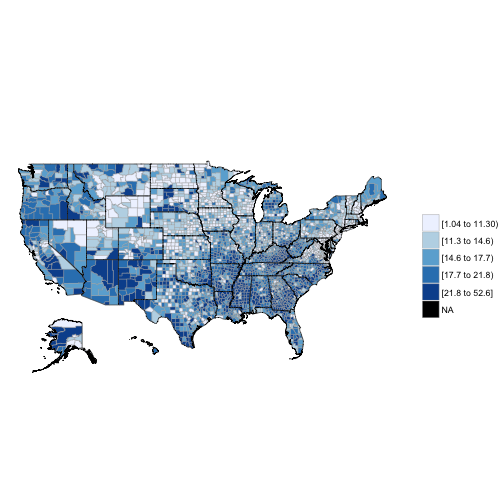In sum, the acsr package:

• Reads formulas directly and extracts any ACS/Census variable
• Provides an automatized and tailored way to obtain indicators and MOEs
• Allows different outputs’ formats (wide and long, csv)
• Provides an easy way to adjust MOEs to different confidence levels
• Includes a variable-by-variable ratio adjustment of standard errors
• Includes the zero-option when computing standard errors for proportions, ratios, and aggregations
• Combines geographic levels flexibly

Last Update: 02/07/2016

]]>
Sebastian Daza
Imputing scales using parcels of items as auxiliary variables2015-10-14T00:00:00+00:002015-10-14T00:00:00+00:00https://sdaza.github.io/blog/2015/imputation-parcelsMultiple imputation of scales generated by several items can be challenging. Fortunately, to impute every single item is not the only solution to the missing data problem. Some practical and theoretically attractive alternative have already been proposed. In this post, I show a simple implementation of what Enders (2010) calls duplicated-scale imputation, a method orginally suggested by Eekhout et al. (2011). By the way, thanks Iris Eekhout for replying my e-mails!

## Procedure

For each scale, I define a number or proportion of items (let’s say p) to create parcels (i.e., average of items although not the whole scale). These parcels are, then, used as auxiliary variables to impute the original scales. There are different ways to define parcels. I implemented a solution in my R package sdazar, see the function rowscore for more details.

The function rowscore selects p items with the least missing data. For each case (row), it computes the parcels using the available information of the selected items. If only one item has information, only that one will be used. If there are more than one item with valid data, it will average all the selected items. If there are no items available in my initial selection, it picks p items from the rest of unselected items to impute the original scale. In this particular example, I create parcels using half of the items:

The reason for using a proportion of the original items is to include as much information as possible, but preventing strong linear dependencies between variables. Ideally, parcels are complete (no missing values). However, in some cases all the items are missing, so parcels can still have missing records (although less than the original scales).

Why not just to use the average of the available items? That solution would implicitly assume that items perfectly correlate with the scale. We know that’s not a good assumption. That is why we worry about creating scales in the first place, right? Using parcels takes advantage of the available information (items with complete information) and the relationship between a portion of items and the scale.

Here I show a simple example using the National Longitudinal Study of Adolescent to Adult Health (Add Health). First, let’s look some descriptives of the variables included in the imputation. I am using information from Wave 1 and 2. The scales/scores I am imputing are depression (19 items) and GPA (4 items). Variables ending with .p are parcels with 1/2 of the items of the original scale.

As expected, the correlation between the scales and parcels is high. GPA has most of the problems. Note that parcels .p still have missing records, although much less than the original scales.

Let’s now impute the scales/scores using the R package MICE.

I adjust the predictor matrix to avoid feedbacks during the imputation (circularity between variables). The trick is to use only complete variables when imputing parcels.

Let’s impute the data!

Below some plots to explore how the imputation goes.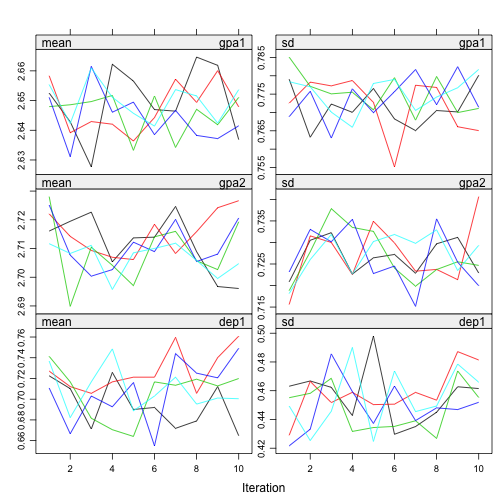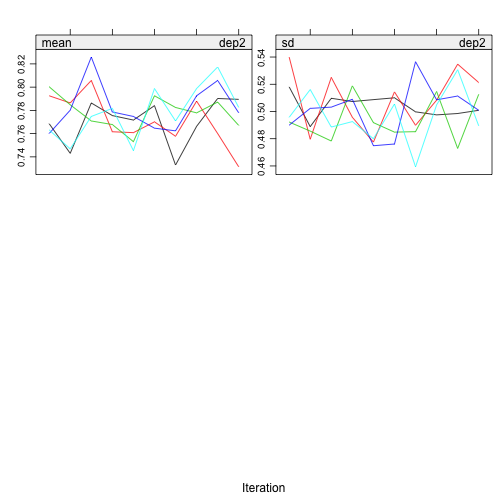I don’t see any problematic pattern. It seems I get a proper solution. The distribution of the variables also looks right.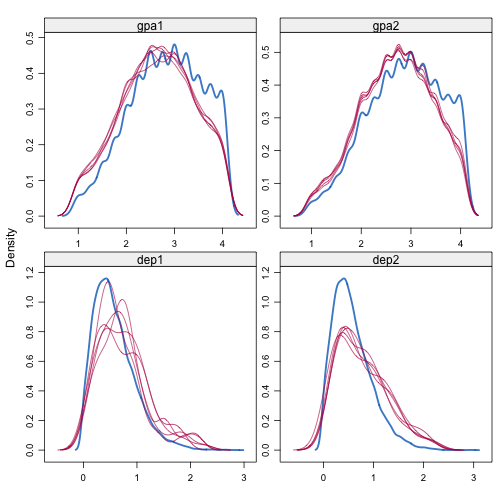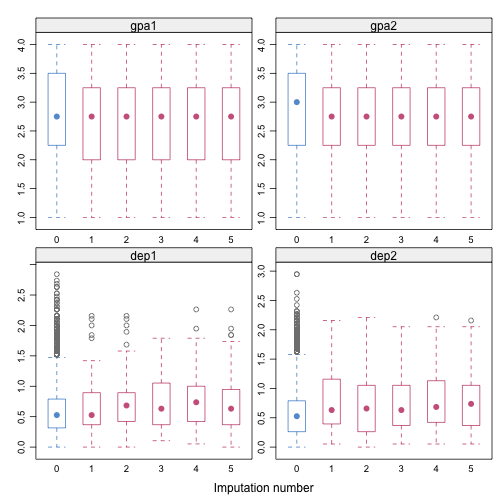Last Update: 06/02/2017

Enders, Craig K. 2010. Applied Missing Data Analysis. The Guilford Press.

Eekhout, Iris, Craig K. Enders, Jos W. R. Twisk, Michiel R. de Boer, Henrica C. W. de Vet, and Martijn W. Heymans. 2015. “Analyzing Incomplete Item Scores in Longitudinal Data by Including Item Score Information as Auxiliary Variables.” Structural Equation Modeling: A Multidisciplinary Journal 22 (4):588-602.

]]>
Simple R package to define sample sizes and MOEs2015-09-30T00:00:00+00:002015-09-30T00:00:00+00:00https://sdaza.github.io/blog/2015/samplerI present a simple R package called sampler. The package defines sample sizes and margins of error (MOE) for proportions, as usually it is needed when designing public opinion surveys. In a previous post, I showed some functions that do mostly the same thing. This new package, though, includes some new features that can be useful when allocating a sample.

## Functions

The packages contains four functions:

• ssize: computes sample size.
• serr: computes MOE.
• astrata: assigns sample sizes to strata.
• serrst: computes MOE for stratified samples.

## Strata allocation: astrata

These examples show how to allocate a sample size into strata. Look at ?astrata in R for definitions of the allocation procedures that are available.

## Combining criteria

We can adjust a bit more:

That’s it. A simple package to do simple calculations.

]]>
Sebastian Daza
Functions for sample size and error2014-01-19T00:00:00+00:002014-01-19T00:00:00+00:00https://sdaza.github.io/blog/2014/samplesizeHere I show two functions in R to define sample sizes and errors of a proportion, taking into account design effect, response rate, finite population correction, and stratification. They are useful when one needs to do these calculations quickly.

Note: I created a package with similar functions. See here.

The inputs are:

• n = sample size
• e = sampling error
• deff = design effect, by default 1 (SRS)
• rr = response rate, by default 1
• N = population size, by default NULL (infinite population)
• cl = confidence level , by default .95
• p = proportion, by default 0.5 (maximum variance of a proportion)
• relative = to estimate relative error, by default FALSE

## serr: sampling error

An example for n = 400 and all inputs at their default values:

The output is rounded to 4 decimals. A more complete example:

• n = 400
• deff = 1.5
• response rate = 80%
• population size = 1000

The sample size (n) has always to be lower than the population (N). It is important to note that the final sample size used to compute the sampling error is:

$n = \frac{N}{deff} * rr$

## ssize: sample size

Let’s get a sample size with an error of .03, a population of 1000 elements, a response rate of 0.80, and an effect design of 1.2:

If the the sample size is bigger than the population because of low response rates or big design effects, the sample size will be fixed to N:

## Working with strata

Finally, we can estimate different sample sizes by strata using vectors or a data frame:

As easy as falling off a log!

]]>
Sebastian Daza
Cohort component projection2013-07-02T00:00:00+00:002013-07-02T00:00:00+00:00https://sdaza.github.io/blog/2013/projectionsI present an example of a cohort component projection using a closed female population (Sweden 1993), taken from Preston et al.’s book (Demography 2001, page 125). I use R and basic matrix algebra to replicate their results. The advantage of this procedure is that allows to compute easily the intrinsic growth rate and age-proportionate distribution of the stable equivalent population. All we need is the population by age at time 0 (from a census), survivorship ratios (from a life table), and age-specific fertility rates.

The data:

As can be seen, the data have five-year-interval age groups, so each projection forward will involve 5 years. The steps are very simple:

1. Project forward the population of each age group (estimation of people alive)
2. Calculate the number of births of each age group based on fertility rates, adjusting by mortality (estimation of children alive)
3. Create a Leslie matrix, and then multiple it by the population vector (population by age at time 0)

### Survivorship ratios

We have to estimate life table survival ratios, that is, proportions of birth cohorts surviving from one age interval to the next in a stationary population. Basically, we are summarizing the mortality experience of different cohorts assuming stationarity. Because census statistics refer to age “last birthday” (rather than exact age), I estimate ratios using $L_x$ (average number of survivors in an age interval) instead of $l_x$.

$S_x = \frac{_5L_x}{_5L_{x-5}}$

I compute the survival ratios using a loop in R. The estimation of the open-ended survival ratio is slightly different but still straightforward:

$\frac{T_{85}}{T_{80}}$

### Number of children

This is the tricky part. Because census statistics refer to age “last birthday”, and we are projecting every 5 years, the estimation of the number of person-years lived by women in each age group consists of the average number of women alive at the beginning and end of the period (assuming a linear change over the period). To take advantage of the Leslie matrix, I define the births in R using a loop as follows:

1/(1+1.05) corresponds to a transformation of age-specific fertility rates (son and daughters) to maternity rates (only daughters), assuming that the ratio of male to female births (SBR) is constant across mothers’ ages. The number of births is also adjusted by the corresponding survival ratio from 0 to 5 years old ($\frac{_5L_0}{5 \times l_0}$), the number 5 goes away due to simplifying).

### Leslie matrix

I construct a Leslie matrix by replacing specific cells of a 18 x 18 matrix (18 age groups) by the vectors defined above (survival ratios and maternity rates):

Here we have the Leslie matrix:

Note that the last survival ratio is repeated in the last column (0.518). This is because the estimation of the open-ended survival ratio is:

$(N_{80} + N_{85}) \times \frac{T_{85}}{T_{80}}$

### Now, let’s do some projections

Using the R multiplication operator for matrices, I do a 5-year projection by simply multiplying the Leslie matrix by the population vector (remember that matrix multiplication is not commutative).

I obtain the same results of the book. Raising this multiplication I can get the projected population of subsequent periods. Because R doesn’t have a power operator for matrices, I define a function called mp to raise matrices (it is not very efficient, but for this example it’s still useful).

Let’s project the initial population for two periods (10 years):

Again, I get the same result of the book. The nice thing of all this is that estimating eigenvalues and eigenvectors, I can obtain the intrinsic growth rate and age-distribution of the “stable equivalent” population. Using the eigen function in R, I can identify the dominant eigenvalue (higher absolute number), and the corresponding eigenvector:

The population is growing but little.

### What about the population momentum?

The population momentum corresponds to the growth of a population after imposing replacement fertility conditions, that is, NRR=1. Thus, the first thing we have to do is to estimate NRR.

We can quickly estimate the intrinsic growth rate using NRR:

Very close to our estimation using cohort component projection. To impose the replacement condition, I just have to divide the first row of the Leslie matrix by NRR.

To get the population momentum we have to project the initial population until the growth is zero (here I raised the matrix 100 times), and then to compute the ratio between the initial population and the non-growing population (stationary).

After imposing the replacement condition, the population grew 1%.

]]>
Sebastian Daza
Looking for variables in R2013-01-29T00:00:00+00:002013-01-29T00:00:00+00:00https://sdaza.github.io/blog/2013/lookvarRecently, I have been working with big databases. After reading their codebooks (usually very long pdf files), I thought it would be useful to have a function to find variable names in R. I wrote a simply function that looks for variable names in data.frame and data.table objects.

Here an example:

Pretty useful, at least for me. You can also use regular expressions to get variables, for instance, something like lookvar(dat, "p5[0-2]_[a-z]+_2").

]]>
Sebastian Daza